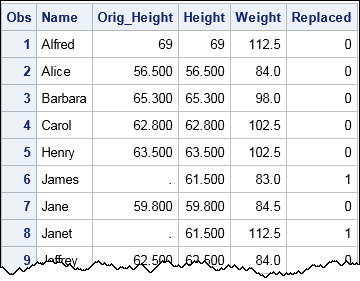Imputing missing data is the act of replacing missing data by nonmissing values. Mean imputation replaces missing data in a numerical variable by the mean value of the nonmissing values. This article shows how to perform mean imputation in SAS. It also presents three statistical drawbacks of mean imputation.

### How to perform mean imputation in SAS

The easiest way to perform mean imputation in SAS is to use PROC STDIZE. PROC STDIZE supports the REPONLY and the METHOD=MEAN options, which tells it to replace missing values with the mean for the variables on the VAR statement. To demonstrate mean imputation, the following statements randomly add missing values to the Sashelp.Class data set. The call to PROC STDIZE then replaces the missing values and creates a data set called IMPUTED that contains the results:

```/* Create "original data" by randomly inserting missing values for some heights */ data Have; set sashelp.class; call streaminit(12345); Replaced = rand("Bernoulli", 0.4); /* indicator variable is 1 about 40% of time */ if Replaced then Height = .; run;   /* Mean imputation: Use PROC STDIZE to replace missing values with mean */ proc stdize data=Have out=Imputed oprefix=Orig_ /* prefix for original variables */ reponly /* only replace; do not standardize */ method=MEAN; /* or MEDIAN, MINIMUM, MIDRANGE, etc. */ var Height; /* you can list multiple variables to impute */ run;   proc print data=Imputed; format Orig_Height Height BESTD8.1; var Name Orig_Height Height Weight Replaced; run;```The output shows that the missing data (such as observations 6 and 8) are replaced by 61.5, which is the mean value of the observed heights. For a subsequent visualization, I have included a binary variable (Replaced) that indicates whether an observation was originally missing. The METHOD= option in PROC STDIZE supports several statistics. You can use METHOD=MEDIAN to replace missing values by the median, METHOD=MINIMUM to replace by the minimum value, and so forth.

### Problems with mean imputation

Most software packages deal with missing data by using listwise deletion: observations that have missing data are dropped from the analysis. Throwing away hard-collected data is painful and can result in a substantial loss of power for statistical tests. Mean imputation, which is easy to implement, enables analysts to use every observation. However, mean imputation has three serious disadvantages that can lead to problems in your statistical analysis. Mean imputation is a univariate method that ignores the relationships between variables and makes no effort to represent the inherent variability in the data. In particular, when you replace missing data by a mean, you commit three statistical sins:

• Mean imputation reduces the variance of the imputed variables.
• Mean imputation shrinks standard errors, which invalidates most hypothesis tests and the calculation of confidence interval.
• Mean imputation does not preserve relationships between variables such as correlations.

These problems are discussed further in my next blog post. Most experts agree that the drawbacks far outweigh the advantages, especially since most software supports modern alternatives to single imputation, such as multiple imputation. My advice: don't use mean imputation if you can use a more sophisticated alternative.

### Epilogue

When I was in college, an actor friend smoked cigarettes. He knew that he should stop, but his addiction was too strong. When he lit up he would recite the following verse and dramatically punctuate the final phrase by blowing a smoke ring:

If you don't smoke, don't start.
If you do smoke, stop.
If you do smoke and won't stop, smoke with style. (*blows smoke ring*)

I don't recommend mean imputation. It is bad for the health of your data. But I can't dissuade from using mean imputation, remember the following verse:

If you don't use mean imputation, don't start.
If you do use mean imputation, stop.
If you do use mean imputation and won't stop, use PROC STDIZE.

The post Mean imputation in SAS appeared first on The DO Loop.